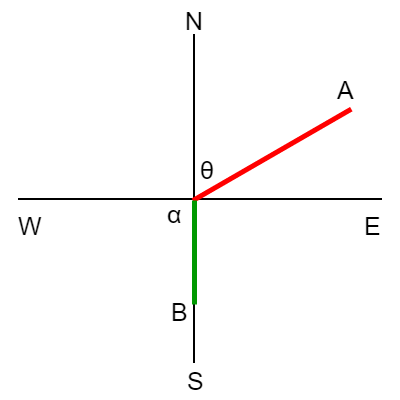# The airspeed of an airplane is 500 mph and its heading is 60^\circ. A wind is blowing from the...

## Question:

The airspeed of an airplane is 500 mph and its heading is {eq}60^\circ. {/eq} A wind is blowing from the south at 40 mph. Find the plane's ground speed and its course.

## Ground Speed and Course.

In aviation, the directions are respect to the y-axis, therefore, when working with vectors, it works differently than when working in other scenarios. Example, for the x-axis we work with the sine of the angle and for the y-axis we work with the cosine of the angle. The decomposition of vectors allows us to find the coordinates of the resulting vector, which would be the ground speed.Data:

We need to find the components of the vectors.

Components of Vector A:

{eq}\begin{align*} A_x&=A\sin\theta \\ A_x&=500\:mph \cdot \sin 60^{\circ} \\ A_x&=433.01\:mph \\ \\ A_y&=A\cos\theta \\ A_y&=500\:mph \cdot \cos 60^{\circ} \\ A_y&=250\:mph \end{align*} {/eq}

Components of Vector B:

{eq}\begin{align*} B_x&=B\sin\alpha \\ B_x&=40\:mph \cdot \sin 180^{\circ} \\ B_x&=0\:mph \\ \\ B_y&=B\cos\alpha \\ B_y&=40\:mph \cdot \cos 180^{\circ} \\ B_y&=-40\:mph \end{align*} {/eq}

The components of the resultant vector are:

{eq}\begin{align*} R_x&=A_x+B_x \\ R_x&=433.01\:mph+0\:mph \\ R_x&=433.01\:mph \\ \\ R_y&=A_y+B_y \\ R_y&=250\:mph-40\:mph \\ R_y&=210\:mph \end{align*} {/eq}

The magnitude of the resultant vector is:

{eq}\begin{align*} R&=\sqrt{R_x^2+R_y^2} \\ R&=\sqrt{\left(433.01\:mph\right)^2+\left(210\:mph\right)^2} \\ R&=481.24\:mph \end{align*} {/eq}

The direction of the resultant vector is:

{eq}\begin{align*} \beta&=\tan^{-1}\left(\frac{R_x}{R_y}\right) \\ \beta&=\tan^{-1}\left(\frac{433.01\:mph}{210\:mph}\right) \\ \beta&=64.12^{\circ} \end{align*} {/eq}

The plane's ground speed is 481.24 mph and its course is N64.12E